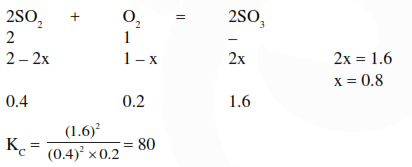Deepak Scored 45->99%ile with Bounce Back Crack Course. You can do it too!

# One mole of

Question:

One mole of $\mathrm{O}_{2}(\mathrm{~g})$ and two moles of $\mathrm{SO}_{2}(\mathrm{~g})$ were heated in a closed vessel of one litre capacity at $1098 \mathrm{~K}$. At equilibrium $1.6$ moles of $\mathrm{SO}_{3}(\mathrm{~g})$ were found. The equilibrium constant $\mathrm{K}_{\mathrm{C}}$ of the reaction would be :-

1. 60

2. 80

3. 30

4. 40

Correct Option: , 2

Solution: